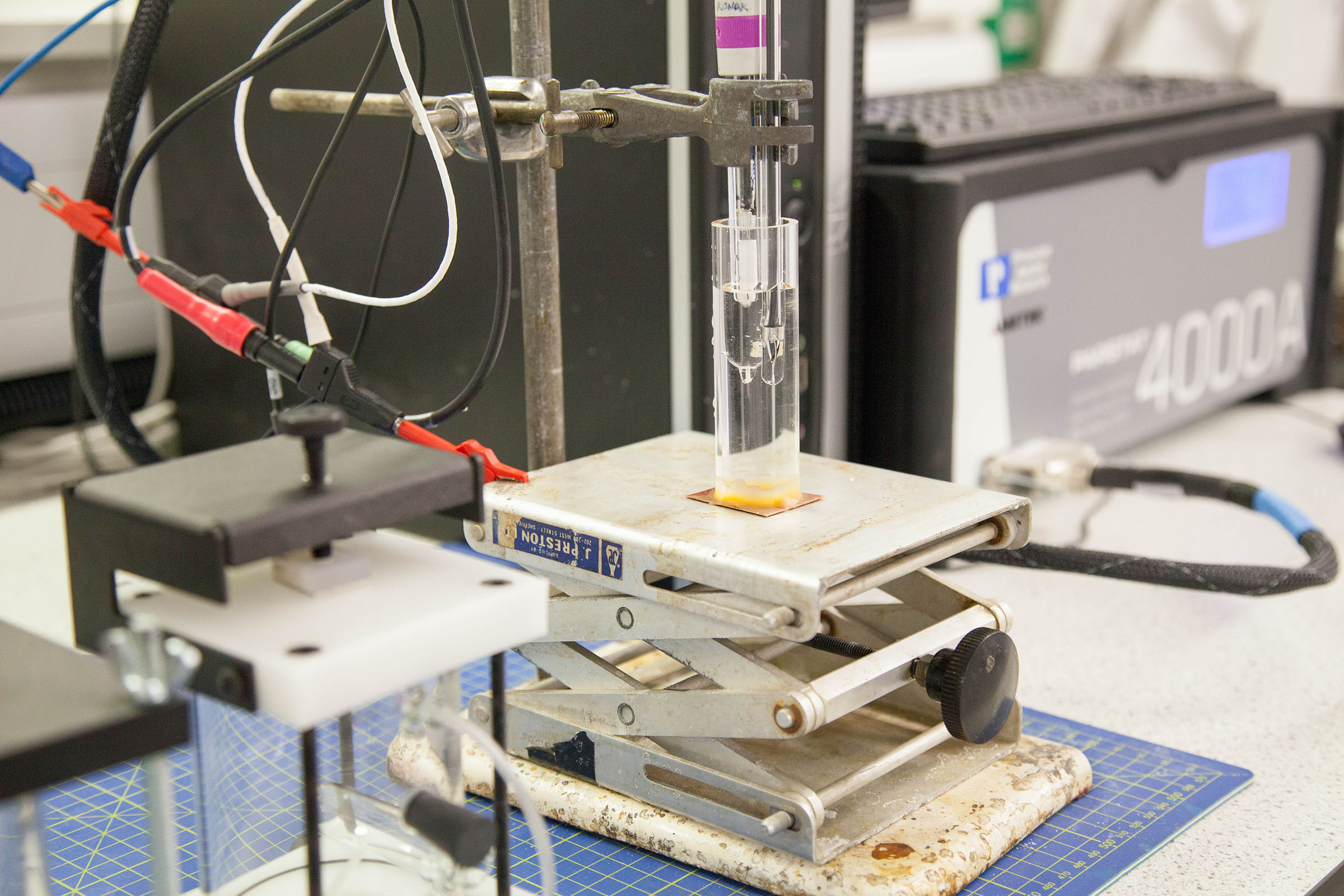DC Electrochemical Techniques | Sheffield Hallam University

Skip to content

# DC Electrochemical Techniques

DC Electrochemical Measurements of Corrosion Rate

Corrosion is a process of deterioration that normally occurs on the surface of the metal contact with the external environment.

For example, in the role of oxygen and water, in the iron or steel will return to a lower energy state such as iron oxide,  and the iron becomes pitted with rust spots. This is a good example of an electrochemical process.

The cell reactions in this mechanism are:

(1) the anodic reaction which generally increases in rate with increasing electrochemical potential e.g.

Fe(s) <==>   Fe+2   +   2 e-1

(2) the  cathodic reaction which generally increases in rate with decreasing electrochemical potential. e.g.

1/2 O2(g)  +  H2O(l)  +  2 e-1 <==>   2 OH-1

The corrosion reaction is a combination of the anodic and cathodic  reactions, which for the two reactions above results in the generation of Ferrous hydroxide.

The overall reaction equation is:

Fe(s)  +  1/2  O2(g) +  H2O(l)  <==>    Fe(OH)2(s)

Polarisation techniques make use of the relationship between the electrochemical

potential of the system and the resultant current. Corrosion current represents both the anodic and cathodic rate of reaction at the natural electrochemical corrosion potential (Ecorr) /. Therefore any change in the external environment which alters the rate of the anodic or cathodic reaction, such as a reduction in oxygen concentration,   will change Ecorr.

Experiments that involve a controlled potential are called potentiostatic experiments, and those that control the current and measure potential are called galvanostatic.

A widely used exploitation of this relationship is linear polarisation resistance (LPR), where a two or three electrode system is submerged in the electrolyte and a small potential is applied.   The resulting current is then measured and from  the relationship between electrochemical potential and resultant current a resistance, called the linear polarisation resistance (LPR)  can be determined from Ohm's Law. Since the resistance is inversely proportional to the rate of corrosion it is now possible to estimate the rate of metal dissolution .A typical electrode arrangement for performing dc or ac electrochemical experiments. The copper foil is the working electrode being studied.

### Cancel event

Are you sure you want to cancel your place on Saturday 12 November?